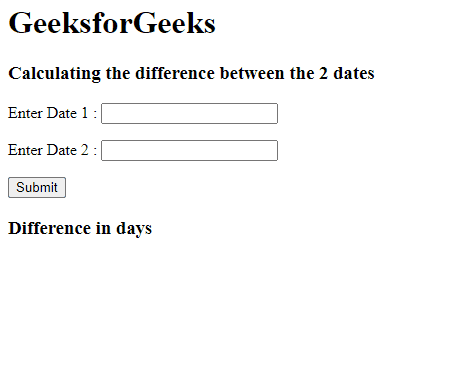GeeksforGeeks App
Open AppBrowser
Continue

# How to get Difference between two Dates in days using jQuery ?

In this article, we will learn how to find the difference between the 2 dates in days using jQuery. Here, we will take the input for the dates using a jquery datepicker.

Considering the given below 2 input Dates, we need to calculate the difference between them which will be returned in a number of days.

```Input : 02/17/2022
02/10/2022
Output: 7```

For this, we will use some functions such as the getTime() method, Date() in the code. We will discuss both methods sequentially.

getTime(): It returns the number of milliseconds since January 1, 1970 00:00:00. This method does not accept any arguments.

Syntax:

`Date.getTime();`

Date(): The Date objects are created with the new Date() constructor. All the arguments passed to the Date() are optional.

Syntax:

`new Date(year, month, day, hours, minutes, seconds, milliseconds)`

jQuery Date Picker: A date-picker of jQuery UI is used to provide a calendar for the user to select the date from a Calendar.

Syntax:

`\$( selector ).datepicker();`

Approach:

• Take two dates as input using datepicker.
• Now, convert them into date types using the Date() method.
• By using getTime() on both dates, we will get the number of milliseconds since January 1, 1970 00:00:00.
• Calculate the absolute difference between the milliseconds of two dates.
• Convert the milliseconds to days by using the following formula
`days = milliseconds / (1000 * 36000 * 24)`

Example: This example illustrates getting the difference between 2 dates in days using jQuery.

## HTML

 `<``html` `lang``=``"en"``>` `<``head``>``    ``<``meta` `charset``=``"utf-8"``>``    ``<``title``>Difference between dates in days``    ``<``link` `href``=``"https://code.jquery.com/ui/1.10.4/themes/ui-lightness/jquery-ui.css"``          ``rel``=``"stylesheet"``>``    ``<``script` `src``=``"https://code.jquery.com/jquery-1.10.2.js"``>``    ````    ``<``script` `src``=``"https://code.jquery.com/ui/1.10.4/jquery-ui.js"``>``    ````    ``<``script``>``        ``\$(function() {``            ``\$("#date1").datepicker();``            ``var date1 = \$("#date1")``        ``});``        ``\$(function() {``            ``\$("#date2").datepicker();``            ``var date2 = \$("#date2")``        ``});``    ` `        ``function func() {``            ``date1 = new Date(date1.value);``            ``date2 = new Date(date2.value);``            ``var milli_secs = date1.getTime() - date2.getTime();``            ` `            ``// Convert the milli seconds to Days ``            ``var days = milli_secs / (1000 * 3600 * 24);``            ``document.getElementById("ans").innerHTML =``            ``Math.round(Math.abs(days));``        ``}``    ````` `<``body``>``    ``<``h1``>GeeksforGeeks``    ``<``h3``>Calculating the difference between the 2 dates``    ` `<``p``>Enter Date 1 :``        ``<``input` `type``=``"text"` `id``=``"date1"``>``    ```  `    ` `<``p``>Enter Date 2 :``        ``<``input` `type``=``"text"` `id``=``"date2"``>``    ```  `    ``<``button` `id``=``"sub"``            ``onclick``=``"func()"``            ``type``=``"button"``> Submit``    ````    ``<``h3` `id``=``"ans"``>Difference in days`````

Output:jQuery Date Calculator

My Personal Notes arrow_drop_up
Related Tutorials